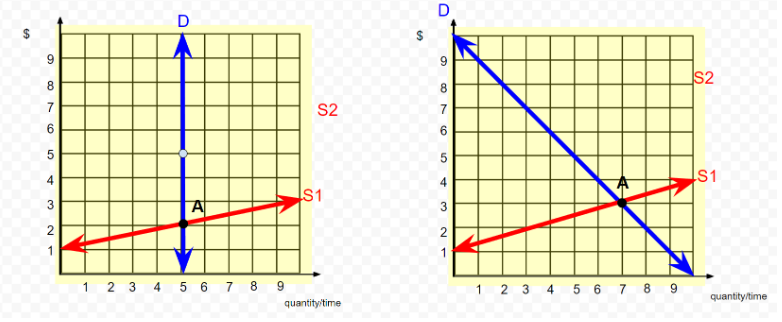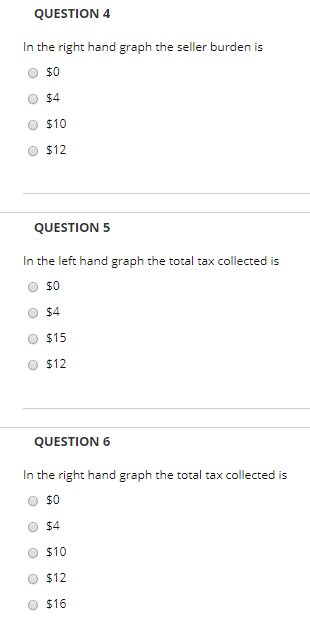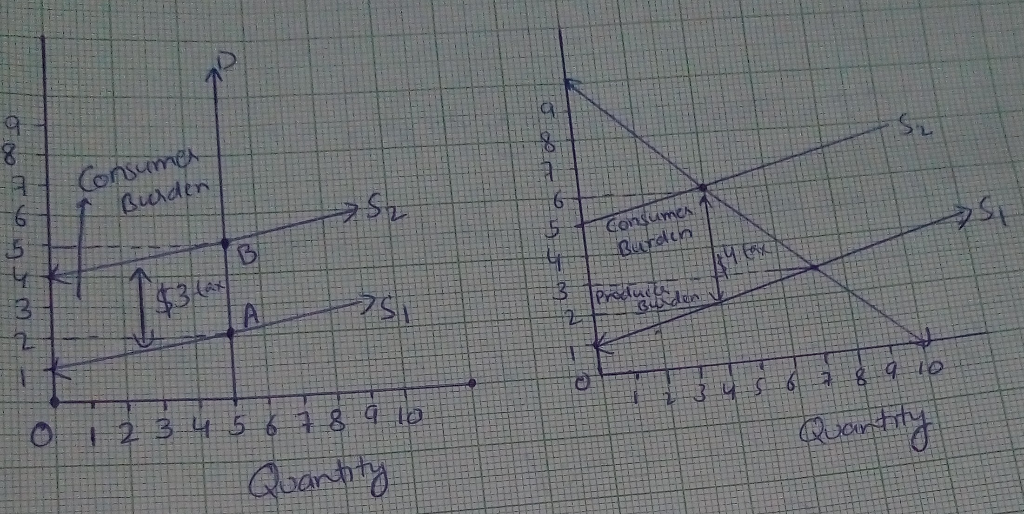# The initial diagram has two panels.The left graph depicts a perfectly inelastic demand curve. The right graph depicts a...

The initial diagram has two panels.The left graph depicts a perfectly inelastic demand curve. The right graph depicts a relatively flat sloped demand curve. The initial equilibrium in each graph is labeled point A. We will impose a \$3 per unit tax in the left graph, and a \$4 per unit tax in the right graph.Make a copy of the graph, then follow the below instructions to draw the graph:

• 1. In the left graph \$3 above and parallel to the original supply curve, draw a new supply curve, put a black dot at the new equilibrium and label it as point B.

• 2. Draw a vertical arrow between the two supply curves, and label it as a \$3 Tax.

• 3. Shade the rectangle representing the rectangle for the tax burden on the buyer.

• 4 In the right graph \$4 above and parallel to the original supply curve, draw a new supply curve, put a black dot at the new equilibrium and label it as point B.

• 5. Draw a vertical arrow between the two supply curves, and label it as a \$4 Tax.

• 6. Shade the rectangles representing the rectangle for the tax burden on the buyer, and the seller, respectively.S2 S2 S1 8 9quantity/time 1 2 3 4 5 6 7 8 9
QUESTION 4 In the right hand graph the seller burden is O \$0 O \$4 O \$10 O \$12 QUESTION 5 In the left hand graph the total tax collected is O \$0 O \$4 O \$15 O \$12 QUESTION 6 In the right hand graph the total tax colected is O \$0 O \$4 O \$10 O \$12 O \$16

Answer -4 Correct option is \$4.

Producer Burden is 4(3-2) = 4

Total tax collected is 5(5-2) = 15

Total tax collected is 4(6-2) = 16.##### Add Answer of: The initial diagram has two panels.The left graph depicts a perfectly inelastic demand curve. The right graph depicts a...
More Homework Help Questions Additional questions in this topic.